# Relation Between The Torque And Angular Momentum Assignment Help

1. Home
2. Physics
3. Rotational Motion
4. Torque
5. Relation Between Torque And Angular Momentum

We know that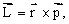on differentiating the equation with respect to time we get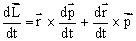=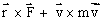[As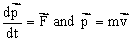]

=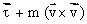[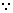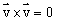]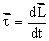## Relation Between The Torque And Angular Momentum Assignment Help By Online Tutoring and Guided Sessions at AssignmentHelp.Net

The above equation is also known as Newton's IInd law of rotation. Hence the time rate of change of angular momentum is equal to net external torque applied an the body.

The simplified form of this equation in the case of pure rotation is,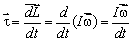=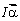This result is the rotational analogue of Newton's second law: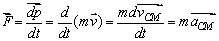### Homework Help For torque and angular momentum

assignmenthelp.net provides best Online Assignment Help service in Physics for all standards. Our Tutor provide their high quality and optimized Tutorial help to fulfill all kind of need of Students.